Courses

# Short Answer Type Questions- Lines and Angles Class 9 Notes | EduRev

## Class 9 : Short Answer Type Questions- Lines and Angles Class 9 Notes | EduRev

The document Short Answer Type Questions- Lines and Angles Class 9 Notes | EduRev is a part of the Class 9 Course Mathematics (Maths) Class 9.
All you need of Class 9 at this link: Class 9

Q.1. If P, Q, and R are three collinear points, then name all the line segments determined by them.

Ans.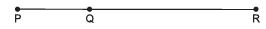We can have the following line segments: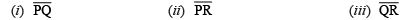Q.2. In the adjoining figure, identify at least four collinear points.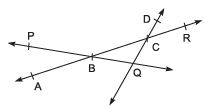Ans. The four collinear points are A, B, C, and R.

Q.3. Find the complement of 36°.
Ans. ∵ 36° + [Complement of 36°] = 90°
⇒ Complement of 36°= 90° - 36° = 54°

Q.4. Find the supplement of 105°.
Ans.  105° + [Supplement of 105°] = 180°
⇒ Supplement of 105° = 180° - 105° = 75°

Q.5. Angles ∠ P and 100° form a linear pair. What is the measure of ∠ P?
Ans.
∵ The sum of the angles of a linear pair equal to 180°.
∴ ∠ P + 100° = 180° ⇒ ∠ P = 180° - 100 = 80°.

Q.6. In the adjoining figure, what is the measure of p?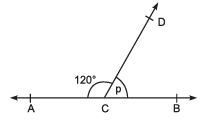Ans. ∵ p and 120° form a linear pair.
∴ p + 120° = 180° ⇒ p = 180° - 120° = 60°

Q.7. In the adjoining figure, AOB is a straight line. Find the value of x.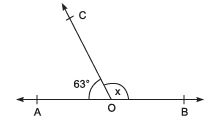Ans. ∵ AOB is a straight line.
∴ ∠AOC + ∠COB = 180°
⇒ 63° + x = 180° ⇒ x = 180° - 63° = 117°

Q.8. In the given figure, AB, CD, and EF are three lines concurrent at O. Find the value of y.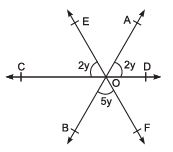Ans. ∵ ∠AOE and ∠BOF and vertically opposite angles.
∴ ∠AOE = ∠BOF = 5y ..... (1)
Now, CD is a straight line,
⇒ ∠COE + ∠EOA + ∠AOD = 180°
⇒ 2y + 5y + 2y = 180° [From (1)]
⇒ 9y = 180°⇒ y = (180°/2)= 20°
Thus, the required value of y is 20°.

Q.9. In the adjoining figure, AB || CD and PQ is transversal. Find x.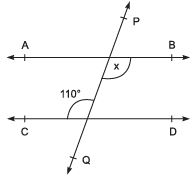Ans. ∵ AB || CD and PQ is a transversal.
∴ ∠ BOQ = ∠ CQP [∵ Alternate angles are equal]
⇒ x = 110° [∵ ∠ CQP = 110°]

Q.10. Find the measure of an angle that is 26° more than its complement.
Ans.
Let the measure of the required angle be x.
∴ Measure of the complement of x° = (90° - x)
⇒ x° - (90° - x) = 26°
⇒ x - 90° + x = 26°
⇒ 2x = 26° + 90° = 116°
⇒ x = (116°/2) = 58°
Thus, the required measure = 58°.

Q.11. Find the measure of an angle if four times its complement is 10° less than twice its complement.
Ans. Let the measure of the required angle be x.
∴ Its complement = (90° - x) and Its supplement = (180° - x)
According to the condition:

4(Complement of x) = 2(Supplement of x) - 10

⇒ 4(90° - x) = 2(180° - x) - 10°
⇒ 360° - 4x = 360° - 2x - 10°
⇒ 4x - 2x = 360° - 360° + 10°
⇒ 2x = 10° ⇒ x = (10°/2)= 5°
Thus, the measure of the required angle is 5°.

Q.12. Two supplementary angles are in the ratio 3:2. Find the angles.
Ans. Let the measure of the two angles be 3x and 2x.
∵ They are supplementary angles.
∴ 3x + 2x = 180°
⇒ 5x = 180° ⇒ x = (180°/5) = 36°
∴ 3x = 3 x 36° = 108° and 2x = 2 x 36° = 72°
Thus, the required angles are 108° and 72°.

Q.13. In the adjoining figure, AOB is a straight line.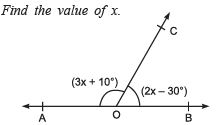Ans. ∵ AOB is a straight line.
∴ ∠ AOC + ∠ BOC = 180°
⇒ (3x + 10°) + (2x - 30°) = 180° [Linear pair]
⇒ 3x + 2x + 10° - 30° = 180°
⇒ 5x - 20° = 180°
⇒ 5x = 180° + 20° = 200° ⇒ x = (200°/5) = 40°
Thus, the required value of x is 40°.

Q.14. In the adjoining figure, find ∠ AOC and ∠ BOD.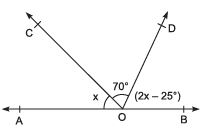Ans. ∵ AOB is a straight line.
∴ ∠AOC + ∠COD + ∠DOB =180°
⇒ x + 70° + (2x - 25°) = 180°
⇒ x + 2x = 180° + 25° - 70°
⇒ 3x = 205° - 70° = 135° ⇒ x = (135°/3) = 45°
∴ ∠ AOC = 45°
⇒ ∠ BOD = 2x - 25° = 2 (45°) - 25° = 90° - 25° = 65°

Q.15. In the adjoining figure, AB || CD. Find the value of x.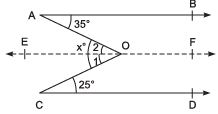Ans. Let us draw EF || AB and passing through point O.
∴ EF || CD and CO is a transversal.
⇒ ∠ 1 = 25° [Alternate angles]
Similarly, ∠ 2 = 35°
Adding, ∠ 1 + ∠ 2 = 25° + 35° ⇒ x = 60°
Thus, the required value of x is 60°.

Offer running on EduRev: Apply code STAYHOME200 to get INR 200 off on our premium plan EduRev Infinity!

## Mathematics (Maths) Class 9

47 videos|324 docs|82 tests

,

,

,

,

,

,

,

,

,

,

,

,

,

,

,

,

,

,

,

,

,

;